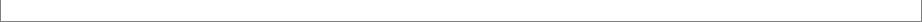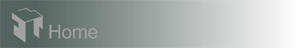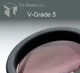Case 2: Convex Lenses in Planetary-Rotation Coater

In this case we examine how a convex-lens surface is coated in the planetary-rotation coater of Case Example 1. We compute the thickness distributions across the diameters of 4 spherical lenses placed along a radius of the fixture. These lenses are 7 cm in diameter and 8 cm in their radius of curvature. For each lens, we compute the thickness at 13 points.

Although the masks correct the thickness distribution perfectly for the flat surface in Case 1, the material deposited on the convex lens surfaces is significantly uneven. In particular, the thickness variation is strongly asymmetric to the centers of the lenses, except the one placed in the middle of the fixture, regardless whether masks are engaged.
To correct the non-center-symmetric thickness variation we may pivot the lenses. To see how this works for the lens that is farthest from the center (represented by points 40 through 52), we may pivot the lens by doing following in "Surface Coated" panel:

1) enter 40 and 52 for first and last selected points, respectively;
2) enter 21.45 and -0.806 for P-X and P-Z (pivoting point), respectively;
3) enter -6.5 for pivoting angle and
4) hit Move button.

Recalculating, you now find the thickness distribution of the lens is now much more symmetric to the center.To see the breadth the technique of repositioning and pivoting substrates can improve thickness uniformity please view Case 3.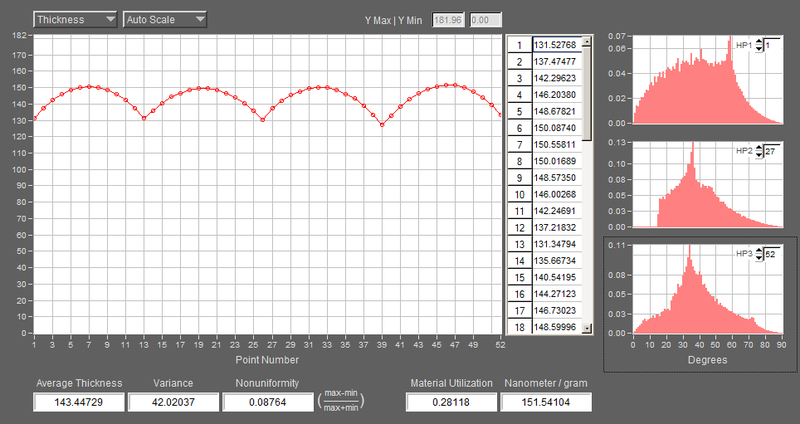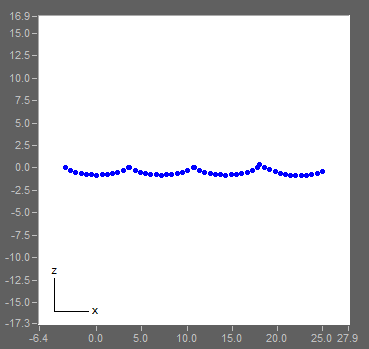Pivoted
-6.5 deg